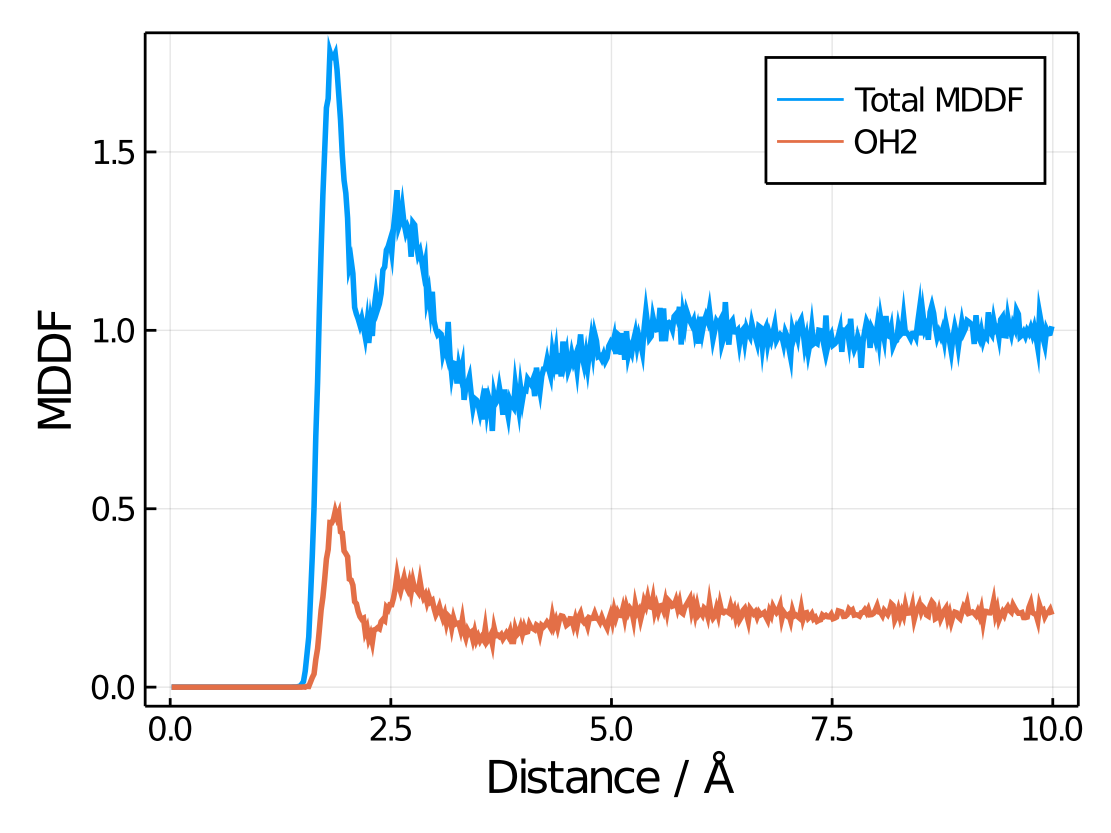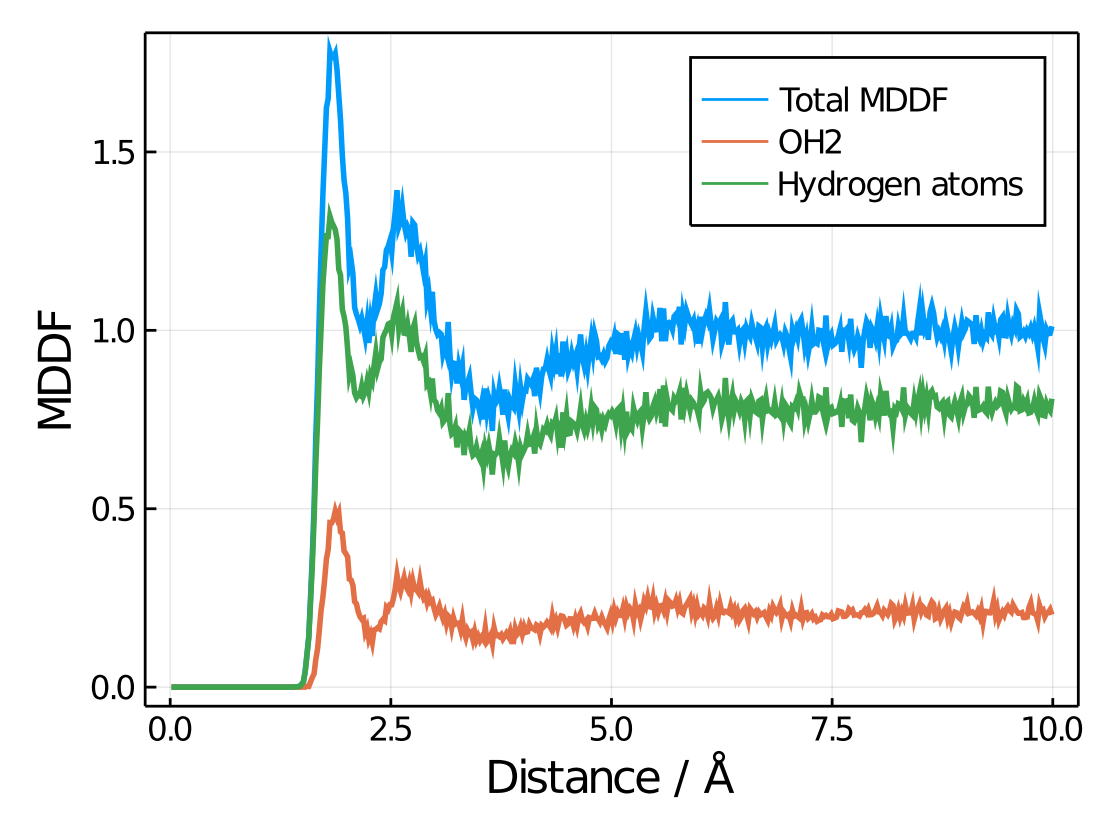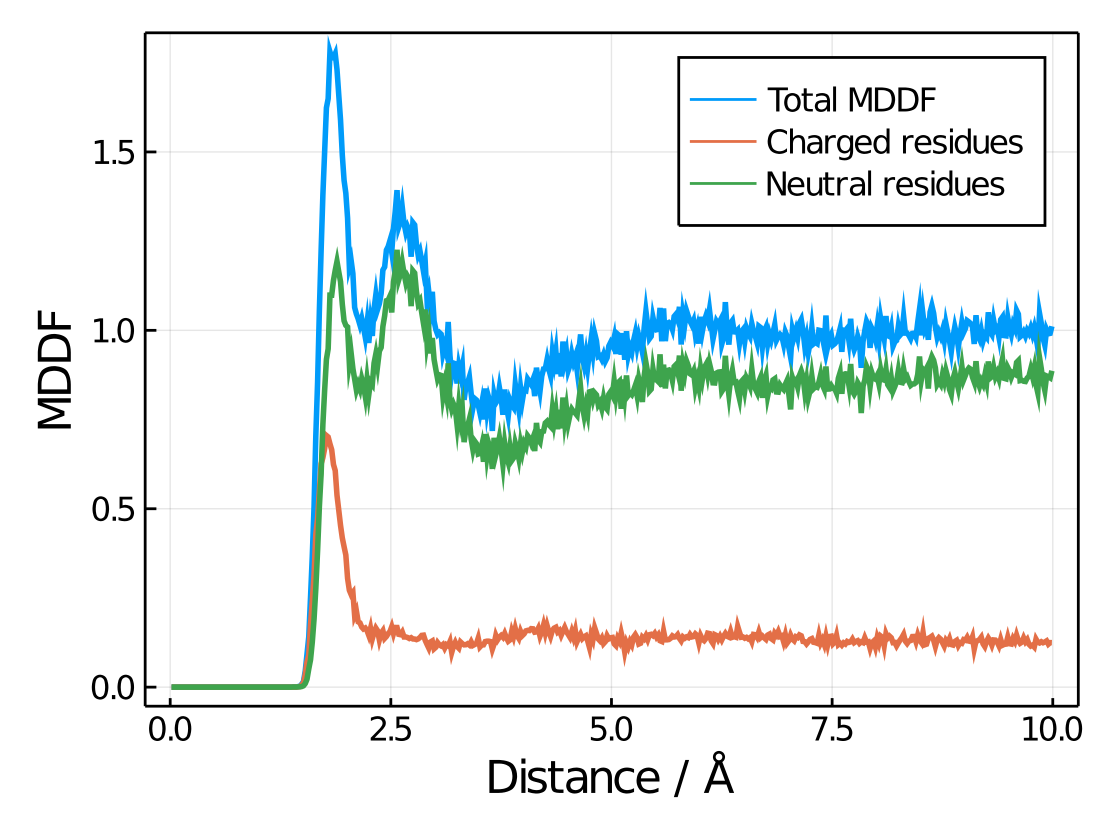# Atomic and group contributions

One of the interesting features of Minimum-Distance distributions is that they can be naturally decomposed into the atomic or group contributions. Simply put, if a MDDF has a peak at a hydrogen-bonding distance, it is natural to decompose that peak into the contributions of each type of solute or solvent atom to that peak.

To obtain the atomic contributions of an atom or group of atoms, the contrib functions are provided. For example, in a system composed of a protein and water, we would have defined the solute and solvent using:

using PDBTools, ComplexMixtures
protein = select(atoms,"protein")
water = select(atoms,"water")
solute = Selection(protein,nmols=1)
solvent = Selection(water,natomspermol=3)

The MDDF calculation is executed with:

trajectory = Trajectory("trajectory.dcd",solute,solvent)
results = mddf(trajectory)

## Atomic contributions in the result data structure

The results data structure contains the decomposition of the MDDF into the contributions of every type of atom of the solute and the solvent. These data is available at the results.solute_atom and results.solvent_atom arrays:

julia> results.solute_atom
50×1463 Array{Float64,2}:
0.0  0.0      0.0  …  0.0  0.0  0.0
0.0  0.0      0.0  …  0.0  0.0  0.0
...
0.0  0.14245  0.0  …  0.0  0.0  0.0
0.0  0.0      0.0  …  0.0  0.0  0.0

julia> results.solvent_atom
50×3 Array{Float64,2}:
0.0        0.0        0.0
0.0        0.0        0.0
...
0.26087    0.26087    0.173913
0.25641    0.0854701  0.170940

Here, 50 is the number of bins of the histogram, whose distances are available at the results.d vector.

It is expected that for a protein most of the atoms do not contribute to the MDDF, and that all values are zero at very short distances, smaller than the radii of the atoms.

The three columns of the results.solvent_atom array correspond to the thee atoms of the water molecule in this example. The sequence of atoms correspond to that of the PDB file, but can be retrieved with:

julia> solvent.names
3-element Array{String,1}:
"OH2"
"H1"
"H2"

Therefore, if the first column of the results.solvent_atom vector is plotted as a function of the distances, one gets the contributions to the MDDF of the Oxygen atom of water. For example, here we plot the total MDDF and the Oxygen contributions:

using Plots
plot(results.d,results.mddf,label="Total MDDF",linewidth=2)
plot!(results.d,results.solvent_atom[:,1],label="OH2",linewidth=2)
plot!(xlabel="Distance / Å",ylabel="MDDF")## Selecting groups by atom names or indexes

To plot the contributions of the hydrogen atoms of water to the total MDDF, we have to select the two atoms, named H1 and H2. The contrib function provides several practical ways of doing that, with or without the use of PDBTools.

The contrib function receives three parameters:

1. The solute or solvent data structure, created with Selection.
2. The array of atomic contributions (here results.solute_atom or results.solvent_atom), corresponding to the selection in 1.
3. A selection of a group of atoms within the molecule of interest, provided as described below.

### Selecting by indexes within the molecule

To select simply by the index of the atoms of the molecules, just provide a list of indexes to the contrib function. For example, to select the hydrogen atoms, which are the second and third atoms of the water molecule, use:

julia> indexes = [ 2, 3 ]
julia> h_contrib = contrib(solvent,R.solvent_atom,indexes)
500-element Array{Float64,1}:
0.0
0.0
⋮
0.7742706465861815
0.8084139794974875

Plotting both the oxygen (index = 1) and hydrogen contributions results in:### Selecting by atom name

The exact same plot above could be obtained by providing lists of atom names instead of indexes to the contrib function:

oxygen = ["OH2"]
o_contrib = contrib(solvent,R.solvent_atom,oxygen)
hydrogens = ["H1","H2"]
h_contrib = contrib(solvent,R.solvent_atom,hydrogens)

The above plot can be obtained with:

using Plots
plot(results.d,results.mddf,label="Total MDDF",linewidth=2)
plot!(results.d,o_contrib,label="OH2",linewidth=2)
plot!(results.d,h_contrib,label="Hydrogen atoms",linewidth=2)
plot!(xlabel="Distance / Å",ylabel="MDDF")

## General selections using PDBTools

More interesting and general is to select atoms of a complex molecule, like a protein, using residue names, types, etc. Here we illustrate how this is done by providing selection strings to contrib to obtain the contributions to the MDDF of different types of residues of a protein to the total MDDF.

For example, if we want to split the contributions of the charged and neutral residues to the total MDDF distribution, we could use to following code. Here, solute refers to the protein.

charged_residues = PDBTools.select(atoms,"charged")
charged_contrib = contrib(solute,R.solute_atom,charged_residues)

neutral_residues = PDBTools.select(atoms,"neutral")
neutral_contrib = contrib(solute,R.solute_atom,neutral_residues)

The charged and neutral outputs are vectors containing the contributions of these residues to the total MDDF. The corresponding plot is:

plot(results.d,results.mddf,label="Total MDDF",linewidth=2)
plot!(results.d,charged_contrib,label="Charged residues",linewidth=2)
plot!(results.d,neutral_contrib,label="Neutral residues",linewidth=2)
plot!(xlabel="Distance / Å",ylabel="MDDF")

Resulting in:Note here how charged residues contribute strongly to the peak at hydrogen-bonding distances, but much less in general. Of course all selection options could be used, to obtain the contributions of specific types of residues, atoms, the backbone, the side-chains, etc.